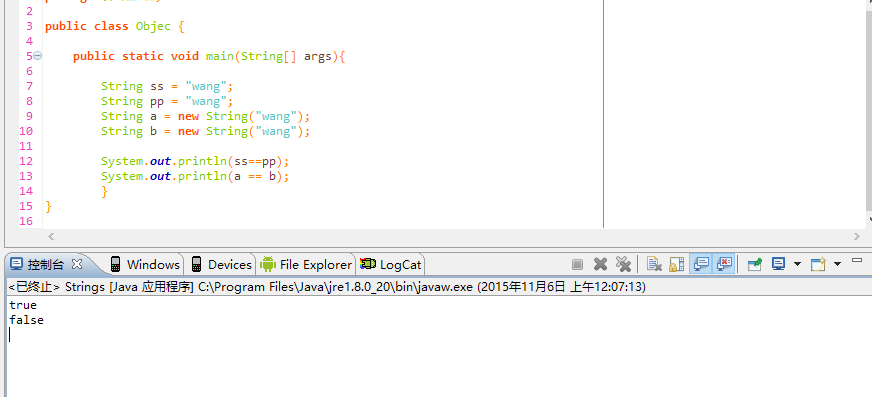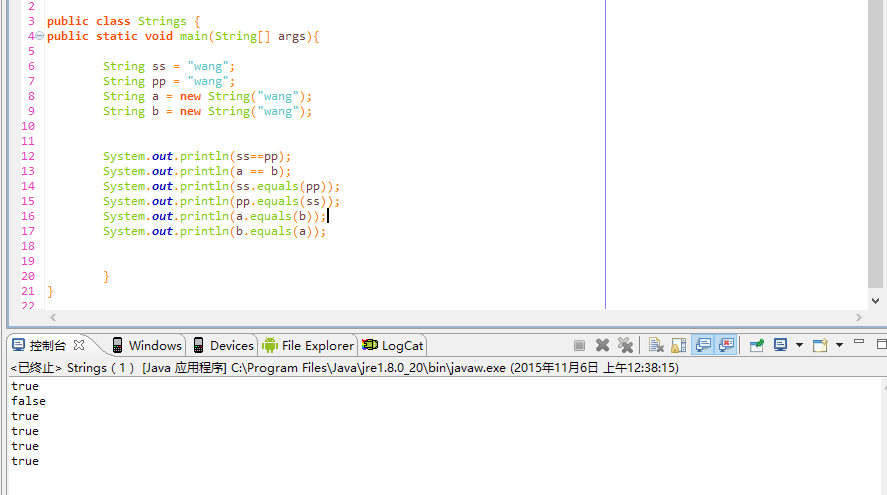# String对象的equals（）与 = =

1. public boolean equals(Object obj) {
2.         return (this == obj);
3.     }

4.     /**
5.      * Creates and returns a copy of this object. The precise meaning
6.      * of "copy" may depend on the class of the object. The general
7.      * intent is that, for any object {@code x}, the expression:
8.      *

9.      *
`			`
10.      * x.clone() != x
11.      * will be true, and that the expression:
12.      *

13.      *
`			`
14.      * x.clone().getClass() == x.getClass()
15.      * will be {@code true}, but these are not absolute requirements.
16.      * While it is typically the case that:
17.      *

18.      *
`			`
19.      * x.clone().equals(x)
20.      * will be {@code true}, this is not an absolute requirement.
21.      *

22.      * By convention, the returned object should be obtained by calling
23.      * {@code super.clone}. If a class and all of its superclasses (except
24.      * {@code Object}) obey this convention, it will be the case that
25.      * {@code x.clone().getClass() == x.getClass()}.
26.      *

27.      * By convention, the object returned by this method should be independent
28.      * of this object (which is being cloned). To achieve this independence,
29.      * it may be necessary to modify one or more fields of the object returned
30.      * by {@code super.clone} before returning it. Typically, this means
31.      * copying any mutable objects that comprise the internal "deep structure"
32.      * of the object being cloned and replacing the references to these
33.      * objects with references to the copies. If a class contains only
34.      * primitive fields or references to immutable objects, then it is usually
35.      * the case that no fields in the object returned by {@code super.clone}
36.      * need to be modified.
37.      *

38.      * The method {@code clone} for class {@code Object} performs a
39.      * specific cloning operation. First, if the class of this object does
40.      * not implement the interface {@code Cloneable}, then a
41.      * {@code CloneNotSupportedException} is thrown. Note that all arrays
42.      * are considered to implement the interface {@code Cloneable} and that
43.      * the return type of the {@code clone} method of an array type {@code T[]}
44.      * is {@code T[]} where T is any reference or primitive type.
45.      * Otherwise, this method creates a new instance of the class of this
46.      * object and initializes all its fields with exactly the contents of
47.      * the corresponding fields of this object, as if by assignment; the
48.      * contents of the fields are not themselves cloned. Thus, this method
49.      * performs a "shallow copy" of this object, not a "deep copy" operation.
50.      *

51.      * The class {@code Object} does not itself implement the interface
52.      * {@code Cloneable}, so calling the {@code clone} method on an object
53.      * whose class is {@code Object} will result in throwing an
54.      * exception at run time.
55.      *
56.      * @return a clone of this instance.
57.      * @exception CloneNotSupportedException if the object's class does not
58.      * support the {@code Cloneable} interface. Subclasses
59.      * that override the {@code clone} method can also
60.      * throw this exception to indicate that an instance cannot
61.      * be cloned.
62.      * @see java.lang.Cloneable
63.      */

1. public String toString() {
2.         return getClass().getName() + "@" + Integer.toHexString(hashCode());
3.     }

4.1. */
2.     public boolean equals(Object anObject) {
3.         if (this == anObject) {
4.             return true;
5.         }
6.         if (anObject instanceof String) {
7.             String anotherString = (String) anObject;
8.             int n = value.length;
9.             if (n == anotherString.value.length) {
10.                 char v1[] = value;
11.                 char v2[] = anotherString.value;
12.                 int i = 0;
13.                 while (n-- != 0) {
14.                     if (v1[i] != v2[i])
15.                             return false;
16.                     i++;
17.                 }
18.                 return true;
19.             }
20.         }
21.         return false;
22.     }

• 博文量
159
• 访问量
989089